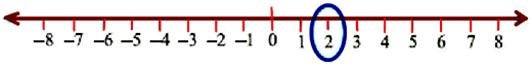# Representation of Integers on a Number Line

Thus, we have integers on the number line as shown in the below figure.

### Consider the following to observe the representation of integers on a number line.

(i) In order to mark +2 on the number line, we move 2 points to the right side of the zero.(ii) In order to mark -6 on the number line, we move 6 points to the left side of the zero.

(iii) In order to mark -1 on the number line, we move 1 point to the left side of the zero.(iv) In order to mark +7 on the number line, we move 7 points to the right side of the zero.(v) In order to mark -4 on the number line, we move 4 points to the left side of the zero.These are the examples to represent integers on a number line.